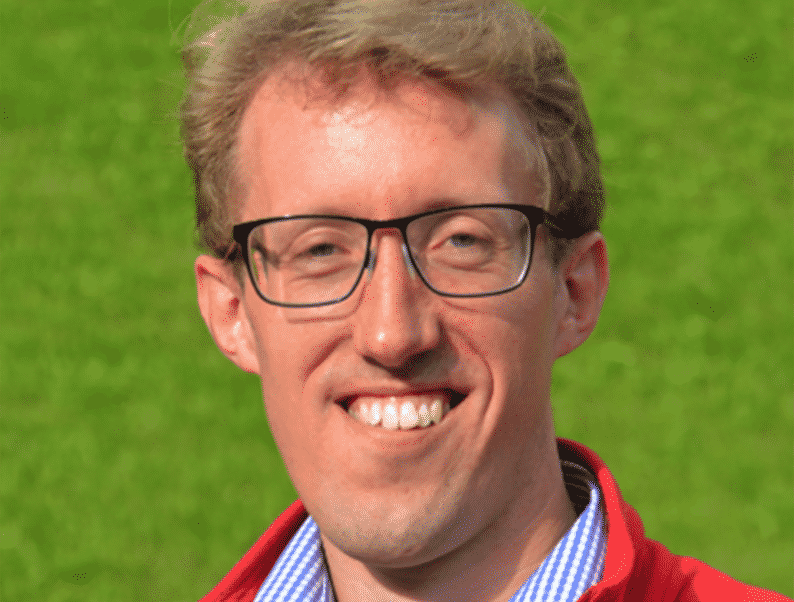Media

## Ian Hewitt

Applied mathematician

I did a maths degree at Oxford but I got increasingly interested in applying maths to other areas of science as I learned more about how seemingly simple equations can explain so many aspects of how things work.  Maths is a good background for studying all sorts of science, as a lot of it is about problem solving and breaking things down to their core essence.   I am now primarily interested in geoscience – I study various aspects of geophysical fluid dynamics and the climate systemIan Hewitt
Applied mathematician

Top